Fajan's Rule, Types of Interactions & Dipole Moment

# Fajan's Rule, Types of Interactions & Dipole Moment Notes | Study Inorganic Chemistry - Chemistry

## Document Description: Fajan's Rule, Types of Interactions & Dipole Moment for Chemistry 2022 is part of Inorganic Chemistry preparation. The notes and questions for Fajan's Rule, Types of Interactions & Dipole Moment have been prepared according to the Chemistry exam syllabus. Information about Fajan's Rule, Types of Interactions & Dipole Moment covers topics like and Fajan's Rule, Types of Interactions & Dipole Moment Example, for Chemistry 2022 Exam. Find important definitions, questions, notes, meanings, examples, exercises and tests below for Fajan's Rule, Types of Interactions & Dipole Moment.

Introduction of Fajan's Rule, Types of Interactions & Dipole Moment in English is available as part of our Inorganic Chemistry for Chemistry & Fajan's Rule, Types of Interactions & Dipole Moment in Hindi for Inorganic Chemistry course. Download more important topics related with notes, lectures and mock test series for Chemistry Exam by signing up for free. Chemistry: Fajan's Rule, Types of Interactions & Dipole Moment Notes | Study Inorganic Chemistry - Chemistry
 1 Crore+ students have signed up on EduRev. Have you?

Application of Fajan’s Rules (i) solubility :

(i) Solubility

• Ag2S is less soluble than Ag2O in H2O because Ag2S is more covalent due to bigger S2– ion.
• Fe(OH)3 is less soluble than Fe(OH)2 in water because Fe+3 is smaller than Fe+2 and thus charge

is more. Fe(OH)3 is more covalent than Fe(OH)2.

(ii) Colour of compounds :

• The colour of some compound can be explained on the basis of polarisation of their bigger negative  ions. For ex : AgCl is white AgBr, Agl, Ag2CO3 are yellow.

The bigger anions are more polarised and hence their electrons get excited by partial absorption of visible light.

• Similarly, SnCl2 is white but SnI2 is black
• PbCl2 is white but PbI2 is yellow.

(iii) Variation of M.P. [M.P. of covalent < M.P. of ionic]

M.P. : BeCl2 < MgCl2 < CaCl2 < SrCl2 < BaCl2
M.P. : CaF2 > CaCl2 > CaBr2 > CaI2

(iv) Nature of oxides :

• Covalent character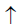acidic naturebasic nature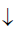Na2O     MgO    Al2O3    SiO2 P2O5 SO3 Cl2O7
Acidic nature Li2O Na2O K2O Rb2O basicNon-Bonding interactions (Intermolecular forces)

Intermolecular attractions hold two or more molecules together. These are weakest chemical forces and can be of following types :
(i) Hydrogen Bonding    (ii) Ion-Dipole interaction   (iii) Van-der Waal’s interaction

(i) Hydrogen Bond

It is electrostatic force of attraction which exist between covalently bonded H-atom of one molecule and the most electronegative element of another molecules.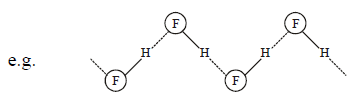Conditions of H-bond

(i) Molecules should have more electronegative atom (F, O, N) linked to the H-atom
(ii) Size of electronegative atom should be smaller.
(iii) A lone pair should be present on electronegative atom.

Nature of H-Bond

It is a very weak electrostatic attraction between positive pole of and lone pair of an electronegative atom which can i.e. F, N or O (But in some cases Cl and C are also found to form H-bonds)

Strength of H-Bond

The strength of H-bond is usually very low (5-10 kJ/mol) but in some cases this value may be as highest 50kJ/mole or more. The strongest H-bonds are fomred by F atoms. D is more positive than H therefore it also form stronger bonds. Besides (F), the Strength of H bonds depends on :
(a) Magnitude of (+) pole of H (For this atom X must be more and more electronegative)
(b) Availability of lone pair on the atom Y.

Types of H-Bonds

(a) Inter Molecular

Exists between the negative and positive ends of different molecules of the same or different substances

(1) In H-F solid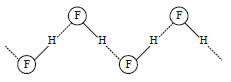The solid KHF2 is an ionic compound consisting of K+ and [HF2] The anion [HF2] consists of H-bonds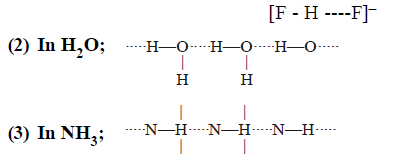(b) Intra molecular

Exists between hydrogen and an electro-negative element of the same molecule. Example are :

(i) O-nitrophenol :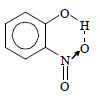(ii) Chloral hydrate :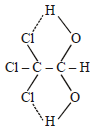(Important example conaisn 2(OH) groups on one C atom)

(a) Ion-dipole attraction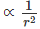Exists between an ion and a polar molecule, its strength depends on (i) size of ion (ii) charge on the ion (iii) dipole moment of the polar molecule.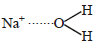This force is responsible of hydration

(b) Dipole-dipole attraction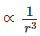Electrostatic attractions between the oppositively charged ends of permanent dipoles. Exists between polar molecules and due to this force gas can be liquified.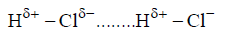(c) Ion-induced dipole attraction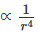Exists between ion and non-polar molecules.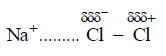(d) Dipole-induced dipole attraction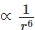Exists between polar and non-polar molecules.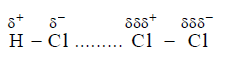(e) Instantaneous dipole-induced dipole attraction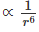Exists among the non-polar molecules like H2, O2, Cl2 etc. in solid or liquid states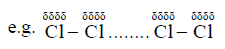Relatrive strength of interactions
Ion-dipole > dipole-dipole > ion-induced dipole > dipole-induced dipole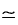instantaneous dipoleinduced
dipole

• Strength of vander waal force ∝ molecular mass
• van der Waal’s force ∝ Boiling point.

Polarity in covalent compounds (Dipole moment)
In diatomic molecule μ = δ x d
A molecule will have a dipole moment of 1 Debye (1D) if charges of 4.8 × 10–10 esu are separated by
a distance of 1 Å.

Thus, 4.8 × 10–10 esu cm = 1 D

but in polyatomic molecule with angle θ, resultant dipole moment is the vector summation of the vector moments.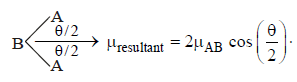where μAB is the vector moment of the side AB.

Also,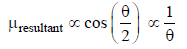Thus, greater the value of θ, smaller the value of μ.

Example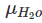(θ =104.50) >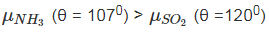Application of dipole moment

(1) To distinguish between polar & non polar molecules: The molecules having some value of dipole moment are polar like HCN, NH3, OCS, PF3, H2O, SO2, NO2, O3 etc.

While those having zero dipole moment are non polar like CO2, BeF2, H2, N2, CH4, SF6, SO3, etc.

(2) To calculate the percentage of ionic character in the polar covalent bond in a given molecule:

Greater is the electronegativity difference between the bonded atoms in a polar molecule, greater is the value of dipole moment.

Percentage of ionic character in A – B bond in AB molecules =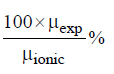(3) To predict the shape of a given molecule: The dipole moment value of a given molecule can be used to predict whether the given molecules has symmetrical or unsymmetrical shape.

1. In a molecule which has symmetrical shape like dumbbell, (e.g. C2, H2, N2, O2, Cl2, Bl2 etc.), linear (e.g. CO2, BeCl2(g), HgCl2), trigonal planar (e.g. BF3, BCl3, SO3 etc.), regular tetrahedral (e.g. CH4, CCl4, CF4 SiF4 etc.), trigonal bipyramidal (e.g. PF5, PCl5(g)), square planar (e.g.XeF4), regular octahedral (e.g. SF6, WF6), pentagonal bipyramidal (IF7) the dipole moments of the vaious bonds present in the molecule cancel each other and hence the net dipole moment of the molecule becomes equal to zero. Thus a molecule which has a symmetrical shape has no (zero) dipole moment.
2. In a molecule which has unsymmetrical shape like angular (e.g. H2O, H2S), trigonal pyramidal (e.g. NH3, NF3, PH3 etc.) the dipole moments of the various bonds present in the molecule are not able to cancel each other and hence the molecule has some value for its dipole moment.

Thus a molecule which has unsymmetrical shape has a certain value of dipole moment.

(4) To Calculate the bond angle between two bonds in a molecule: The angle subtended by two axes of overlaps of orbitals or bond is called bond angle.
Net dipole moment of the molecule =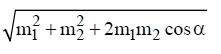in which m1 and m2 are the bond moments of various bonds present in the molecule.

Examples:
(i) Symmetrical molecules without lone pair of electrons will have μ = 0.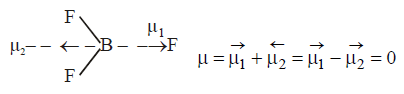In this case resultant μ2 of H - B - H on the left is cancelled by μ of the right.
O = C = O            θ =1800            μ = 0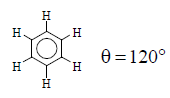, planar , μ = 0

(ii) Symmetrical molecules with lone pair do not have dipole moments equal to zero.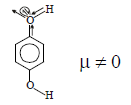(iii) Dipole moment in ring systems

if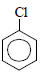Dipole moment = μ, Then, by using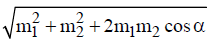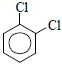Dipole moment = √3 μ , α = 60°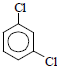Dipole moment = μ , α = 120°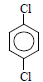Dipole moment = 0 , α = 180°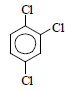Dipole moment = μ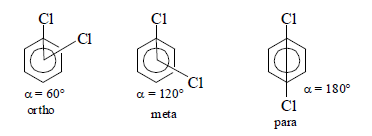Theoretical μ = 6.30D       μ = 3.80D         μ = 0.00D

Experimental μ = 6.00D    μ = 3.79           μ = 0.00D
In this case μ(o-isomer) > μ(m-isomer) > μ(p-isomer) but experimental value of o-isomer < theoretical value.

This is due to dipole-dipole repulsion in o-isomer that increases bond angle greater than 60° and μ decreases.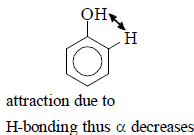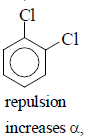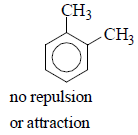(iv) Dipole moment in Geometrical Isomers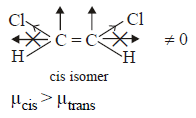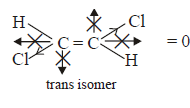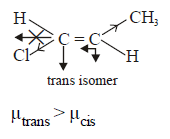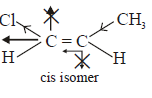(v) Dipole moment in conjugated system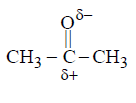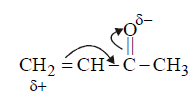As charge separation increases in conjugated systems, dipole moment is extremely high in them.

The document Fajan's Rule, Types of Interactions & Dipole Moment Notes | Study Inorganic Chemistry - Chemistry is a part of the Chemistry Course Inorganic Chemistry.
All you need of Chemistry at this link: Chemistry

## Inorganic Chemistry

36 videos|71 docs|16 tests
 Use Code STAYHOME200 and get INR 200 additional OFF

## Inorganic Chemistry

36 videos|71 docs|16 tests

Track your progress, build streaks, highlight & save important lessons and more!

,

,

,

,

,

,

,

,

,

,

,

,

,

,

,

,

,

,

,

,

,

,

,

,

;